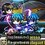# Definite Integral of $x^{x}$ from $x=0$ to $x=1$

$\text{Prove the following integral:}$

$\int_{0}^{1}{x^xdx}=\ \frac{1}{{\ 1}^1\ }-\frac{1}{{\ 2}^2\ }+\frac{1}{{\ 3}^3\ }-\frac{1}{{\ 4}^4\ }+\cdots+\frac{{\ \left(-1\right)}^{n-1}\ }{n^n}+\cdots$





By expanding $x^{x}$ as a series:

$x^x=e^{\ln\left(x^x\right)}=e^{x\ln\left(x\right)}=\sum_{n=0}^{\infty}\frac{{\left(x\ln\left(x\right)\right)}^n}{n!}$

$\int_{0}^{1}{x^xdx}=\int_{0}^{1}{\sum_{n=0}^{\infty}\frac{{\left(x\ln\left(x\right)\right)}^n}{n!}dx}=\sum_{n=0}^{\infty}{\frac{1}{n!}\int_{0}^{1}{x^n\left(\ln\left(x\right)\right)^ndx}}$



Make the substitution of $u=-\ln (x)$, following by $t=u(n+1)$ and you get that:



$\int_{0}^{1}{x^n\left(\ln\left(x\right)\right)^ndx}=\int_{\infty}^{0}{\left(e^{-u}\right)^n\left(-u\right)^n\left(-e^{-u}\right)du}=\left(-1\right)^n\int_{0}^{\infty}{e^{-u\left(n+1\right)}u^ndu}$

$\left(-1\right)^n\int_{0}^{\infty}{e^{-u\left(n+1\right)}u^ndu}=\left(-1\right)^n\int_{0}^{\infty}{e^{-t}\frac{t^n}{\left(n+1\right)^{n+1}}dt}=\frac{\left(-1\right)^n}{\left(n+1\right)^{n+1}}\int_{0}^{\infty}{e^{-t}t^ndt}$



Using the gamma function:

$\int_{0}^{\infty}{e^{-t}t^ndt}=\Gamma(n-1)=n!$



Therefore:

\begin{aligned} \int_{0}^{1}{x^xdx} &= \sum_{n=0}^{\infty}{\frac{1}{n!}\int_{0}^{1}{x^n\left(\ln\left(x\right)\right)^ndx}} \\ &= \sum_{n=0}^{\infty}{\frac{{\left(-1\right)}^n}{n!}\int_{0}^{\infty}{e^{-u\left(n+1\right)}u^ndu}} \\ & = \sum_{n=0}^{\infty}{\frac{\left(-1\right)^n\ }{n!\left(n+1\right)^{n+1}}\int_{0}^{\infty}{e^{-t}t^ndt}} \\ & = \sum_{n=0}^{\infty}\frac{{n!\left(-1\right)}^n\ }{n!\left(n+1\right)^{n+1}} \\ & = \sum_{n=1}^{\infty}\frac{\left(-1\right)^{n-1}\ }{n^n} \\ & = \frac{1}{{\ 1}^1\ }-\frac{1}{{\ 2}^2\ }+\frac{1}{{\ 3}^3\ }-\frac{1}{{\ 4}^4\ }+\cdots+\frac{{\ \left(-1\right)}^{n-1}\ }{n^n}+\cdots \ \ \ (\text{Proven!}) \\ \end{aligned}

$\/extract_itex] $\\$ $\text{Bonus:}$ $x^{-x}=e^{\ln\left(x^{-x}\right)}=e^{{-x}\ln\left(x\right)}=\sum_{n=0}^{\infty}\frac{{(-1)^{n}\left(x\ln\left(x\right)\right)}^n}{n!}$ $\int_{0}^{1}{x^{-x}dx}=\int_{0}^{1}{\sum_{n=0}^{\infty}\frac{{(-1)^{n}\left(x\ln\left(x\right)\right)}^n}{n!}dx}=\sum_{n=0}^{\infty}{\frac{(-1)^{n}}{n!}\int_{0}^{1}{x^n\left(\ln\left(x\right)\right)^ndx}}$ $\\$ \begin{aligned} \int_{0}^{1}{x^{-x}dx} &= \sum_{n=0}^{\infty}{\frac{(-1)^{n}}{n!}\int_{0}^{1}{x^n\left(\ln\left(x\right)\right)^ndx}} \\ &= \sum_{n=0}^{\infty}{\frac{{(-1)^{n}\left(-1\right)}^n}{n!}\int_{0}^{\infty}{e^{-u\left(n+1\right)}u^ndu}} \\ & = \sum_{n=0}^{\infty}{\frac{1}{n!\left(n+1\right)^{n+1}}\int_{0}^{\infty}{e^{-t}t^ndt}} \\ & = \sum_{n=0}^{\infty}\frac{n!}{n!\left(n+1\right)^{n+1}} \\ & = \sum_{n=1}^{\infty}\frac{1}{n^n} \\ & = \frac{1}{{\ 1}^1\ }+\frac{1}{{\ 2}^2\ }+\frac{1}{{\ 3}^3\ }+\frac{1}{{\ 4}^4\ }+\cdots+\frac{1}{n^n}+\cdots \end{aligned}Note by Jian Hau Chooi 2 years, 10 months ago This discussion board is a place to discuss our Daily Challenges and the math and science related to those challenges. Explanations are more than just a solution — they should explain the steps and thinking strategies that you used to obtain the solution. Comments should further the discussion of math and science. When posting on Brilliant: • Use the emojis to react to an explanation, whether you're congratulating a job well done , or just really confused . • Ask specific questions about the challenge or the steps in somebody's explanation. Well-posed questions can add a lot to the discussion, but posting "I don't understand!" doesn't help anyone. • Try to contribute something new to the discussion, whether it is an extension, generalization or other idea related to the challenge. • Stay on topic — we're all here to learn more about math and science, not to hear about your favorite get-rich-quick scheme or current world events. MarkdownAppears as *italics* or _italics_ italics **bold** or __bold__ bold - bulleted- list • bulleted • list 1. numbered2. list 1. numbered 2. list Note: you must add a full line of space before and after lists for them to show up correctly paragraph 1paragraph 2 paragraph 1 paragraph 2 [example link](https://brilliant.org)example link > This is a quote This is a quote  # I indented these lines # 4 spaces, and now they show # up as a code block. print "hello world" # I indented these lines # 4 spaces, and now they show # up as a code block. print "hello world" MathAppears as Remember to wrap math in $$ ... $$ or \[ ...  to ensure proper formatting.
2 \times 3 $2 \times 3$
2^{34} $2^{34}$
a_{i-1} $a_{i-1}$
\frac{2}{3} $\frac{2}{3}$
\sqrt{2} $\sqrt{2}$
\sum_{i=1}^3 $\sum_{i=1}^3$
\sin \theta $\sin \theta$
\boxed{123} $\boxed{123}$

## Comments

Sort by:

Top Newest

Nice use of gamma function .

- 2 years, 10 months ago

Log in to reply

With that bonus:

If $\displaystyle\int_{0}^{1}f(x)dx =\large \displaystyle\sum_{x=1}^{\infty}f(x)$

then $f(x)=x^{-x}$.

- 2 years, 10 months ago

Log in to reply

Wow! Is this the only solution?

- 2 years, 10 months ago

Log in to reply

Interesting question! For starters, $\displaystyle\lim_{x\rightarrow \infty}f(x)=0$

- 2 years, 10 months ago

Log in to reply

×

Problem Loading...

Note Loading...

Set Loading...